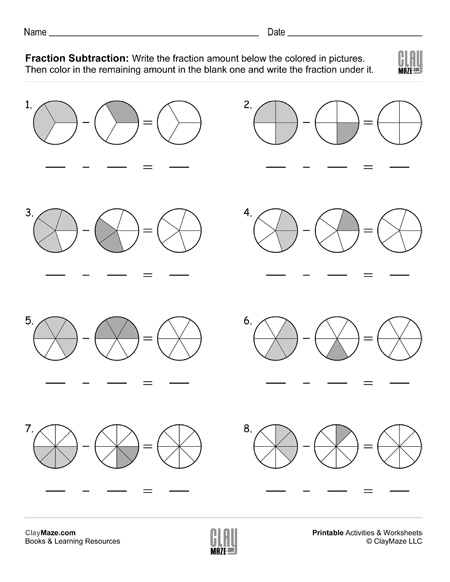Fraction Subtraction with Same Denominators (Set 1)

Subtracting fractions worksheet – Write the fraction amount below the picture. Subtract the fractions and color in the remaining amount in the picture.